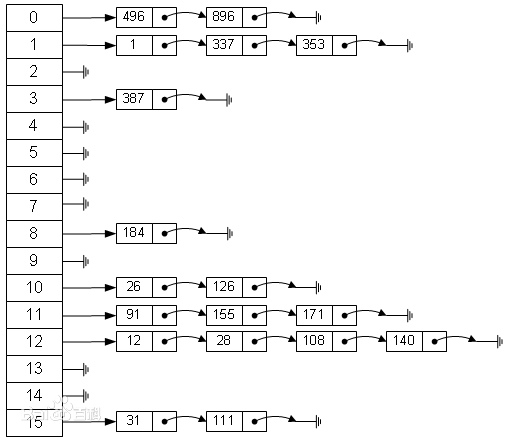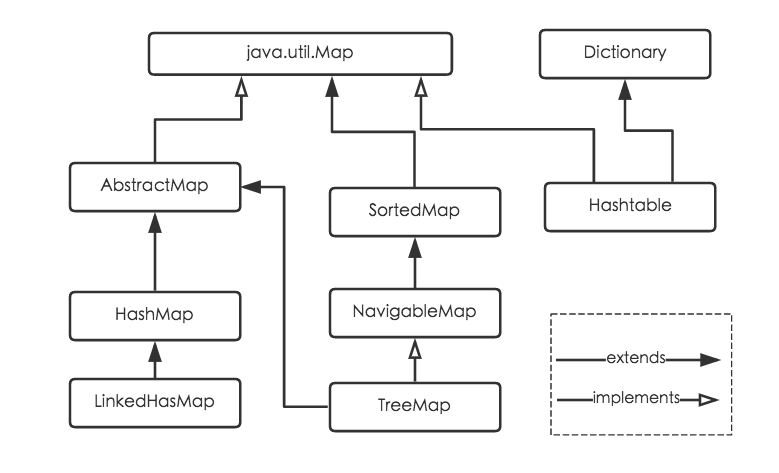# 手把手教你锤面试官 01——HashMap 面试全攻略﻿

0.HashMap的结构是怎么样的？

1.HashMap为什么线程不安全？

2.HashMap线程不安全在程序中的体现是什么？

3.既然HashMap线程不安全，为什么我们还经常用它？

4.怎么样可以让HashMap线程安全？

﻿

0.0回答

﻿﻿

1.1回答

HashMap中put操作的主函数，如果没有hash碰撞则会直接插入元素。如果线程A和线程B同时进行put操作，刚好这两条不同的数据hash值一样，并且该位置数据为null，所以这线程A、B都会进入下面代码的第6行代码中。

﻿

final V putVal(int hash, K key, V value, boolean onlyIfAbsent,                   boolean evict) {        Node<K,V>[] tab; Node<K,V> p; int n, i;        if ((tab = table) == null || (n = tab.length) == 0)            n = (tab = resize()).length;        if ((p = tab[i = (n - 1) & hash]) == null) // 如果没有hash碰撞则会直接插入元素            tab[i] = newNode(hash, key, value, null);        else {            Node<K,V> e; K k;            if (p.hash == hash &&                ((k = p.key) == key || (key != null && key.equals(k))))                e = p;            else if (p instanceof TreeNode)                e = ((TreeNode<K,V>)p).putTreeVal(this, tab, hash, key, value);            else {                for (int binCount = 0; ; ++binCount) {                    if ((e = p.next) == null) {                        p.next = newNode(hash, key, value, null);                        if (binCount >= TREEIFY_THRESHOLD - 1)                             treeifyBin(tab, hash);                        break;                    }                    if (e.hash == hash &&                        ((k = e.key) == key || (key != null && key.equals(k))))                        break;                    p = e;                }            }            if (e != null) {                 V oldValue = e.value;                if (!onlyIfAbsent || oldValue == null)                    e.value = value;                afterNodeAccess(e);                return oldValue;            }        }        ++modCount;        if (++size > threshold)            resize();        afterNodeInsertion(evict);        return null;}

2.2回答

﻿

3.3回答

﻿

HashTable类是线程安全的，它使用synchronize来做线程安全，全局只有一把锁，在线程竞争比较激烈的情况下hashtable的效率是比较低下的。因为当一个线程访问hashtable的同步方法时，其他线程再次尝试访问的时候，会进入阻塞或者轮询状态，进而导致线程挂起。当你在多线程场景下使用Map，虽然不能使用HashMap但也尽量别使用HashTable。其实我个人觉得这个类可以废掉了。

﻿

﻿

﻿﻿

4.4回答

﻿

﻿## 评论